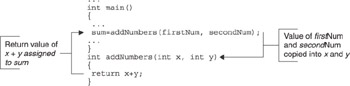# Returning a Value from a Function

Arguments are used to pass values to a called function. A return value can be used to pass a value from a called function back to the function that called it.

For example, in the previous program the function addNumbers had three arguments, the first two being the numbers to be added, the third being their sum. The following program modifies the previous one by eliminating the third argument, but adding a return value to the function:

` #include <iostream> using namespace std; int addNumbers(int, int);  int main () {  int firstNum, secondNum, sum = 0;  cout << "Enter first number: ";  cin >> firstNum;  cout << "Enter second number: ";  cin >> secondNum;  sum = addNumbers (firstNum, secondNum);  cout << firstNum << " + " << secondNum << " = " << sum;  return 0; } int addNumbers (int x, int y) {  return x + y; } `

The sample input and output may be the same as in the previous program:

` Enter first number: 3 Enter first number: 6 3 + 6 = 9 `

The return value is added by indicating its data type, here an int, in front of the function name in both the function prototype and header:

` int addNumbers(int, int);  int addNumbers (int x, int y) `

The function call is on the right side of the assignment operator. To the left of the assignment operator is a variable of the same data type as the return value of the function. The concept is that the return value from the function call is assigned to the variable sum on the left side of the assignment operator.

` sum = addNumbers (firstNum, secondNum); `

The body of the called function has the return keyword followed by a value of the data type compatible with the function prototype and header, here int. The function s return value is the value that follows the return keyword, here the sum of the two arguments:

` return x + y; `

That sum of x + y then is assigned to the variable sum in main.

Figure 9-3 shows the order of execution graphically.Figure 9-3: The order of execution of the return value of a function

It is common that a function returning a value is called on the right side of an assignment operator with a variable on the left side of the assignment operator to capture the return value. However, this is not required. In the program, the variable sum was not necessary. Instead of the lines

` sum = addNumbers (firstNum, secondNum);  cout << firstNum << " + " << secondNum << " = " << sum; `

the return value could have been displayed as:

` cout << firstNum << " + " << secondNum << " = "   << addNumbers (firstNum, secondNum); `

The only difference is that once this cout statement completes, the return value of the function cannot be used in later statements since it was not stored in a variable. In this program, that is not a problem because the return value is not used again. However, if you are going to use a return value more than once, it s generally a good idea to store that return value in a variable. This is typically done by calling the function on the right side of an assignment operator with a variable on the left side of the assignment operator to capture the return value.

While multiple values can be passed to a function as arguments, at this point, multiple values cannot be returned from functions using the data types we have covered so far. This will change when we cover arrays in the next chapter, and structures and classes in later chapters.C++ Demystified(c) A Self-Teaching Guide
ISBN: 72253703
EAN: N/A
Year: 2006
Pages: 148